Mechanics

# Bernoulli equation derivation with examples and applications

Bernoulli equation is defined as the sum of pressure, the kinetic energy and potential energy per unit volume in a steady flow of an incompressible and nonviscous fluid remains constant at every point of its path.”Atomizer and ping pong ball in Jet of air are examples of Bernoulli’s theorem, and the Baseball curve, blood flow are few applications of Bernoulli’s principle.

## Bernoulli’s principle formula

P+ ρgh +1/2 ρv2 =constant

Bernoulli’s equation, which is a fundamental relation in fluid mechanics, is not a new principle but is derivable from the basic laws of Newtonian mechanics. We find it convenient to derive it from the work-energy theorem, for it is essentially a statement of the work-energy theorem for fluid flow.

## Bernoulli equation derivation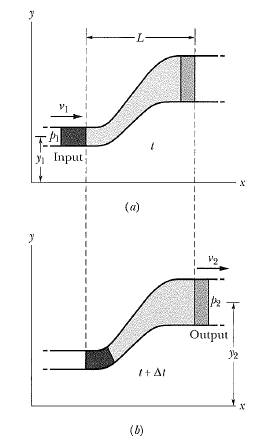Consider the steady, incompressible, nonviscous, and irrotational flow of a fluid through the pipeline or tube of the flow as shown in the figure. The portion of the pipe shown in the figure has a uniform cross-section A1, at the left. It is horizontal there at an elevation y1 above some reference level. It gradually widens and rises and at the right has a uniform cross-section A2. It is horizontal there at an elevation y2. At all points in the narrow apart of the pipe the pressure is p1 and the speed v1; at all points in the wide portion the pressure is p2 and the speed is v2.

The work-energy theorem states: The work done by the resultant force acting on a system is equal to the change in kinetic energy of the system. In the figure, the forces that do work on the system, assuming that we can neglect viscous forces, are the pressure forces p1A1 and p2A2 that act on the left and right-hand ends of the system, respectively, and the force of gravity. As fluid flows through the pipe the net effect. We can find the work W done on the system by the resultant force as follows:

1. The work is done on the system by the pressure force p1A1 and p1A1Δl1.
2. The work done on the system by the pressure force p2A2 is p2A2Δl2 . Note that it is negative because the force acts in a direction opposite to the horizontal displacement.
3. The work done on the system by gravity is associated with lifting the fluid element from height y1 to height y2 and is -Δmg(y2 – y1) in which Δm is the mass of fluid in either area. This contribution is also negative because the gravitational force acts in a direction opposite to the vertical displacement.

The network W done on the system by all the forces is found by adding these three terms, or

W = p1A1Δl1 – p2A2Δl2 -Δmg(y2 – y1)

Now A1Δl1 and A2Δl2 is the volume of the fluid element which we can write as Δm in which ρ  is the constant fluid density. Recall that the two-fluid elements have the same mass, so in a setting:

A1Δl1 = A2Δl2

we have assumed the fluid to be incompressible. With this assumption we have

W=(p1 –p2)(Δm/ρ – Δmg(y2-y2) ………….(1)

The change in kinetic energy of the fluid element is: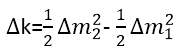From the work-energy theorem, W=Δk,we then have: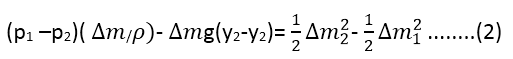After canceling the common factor of m, we can be rearranged to read: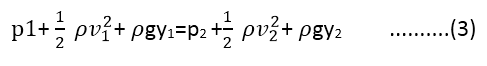Since the subscript 1 and 2 refer to any two locations along the pipeline, we can drop the subscript and write: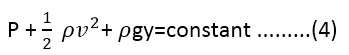Equation (4) is called Bernoulli’s equation for steady, incompressible, nonviscous, and irrotational flow. It was first presented by Daniel Bernoulli in his Hydrodynamica in 1738.

### Applications of Bernoulli’s principle

• venturi tube
• Baseball Curve
• Sailboats
• Torricelli’s theorem
• Blood Flow

Watch video also:

Related topics:

### One Comment

1.mounika says:

this is very useful to us